# 风险控制：信用评分卡模型

## 什么是信用评分卡模型？

• 判断快速：系统只需要按照评分卡逐项打分，最后通过相应的公式计算出总分，即可准确判断出是否为客户授信以及额度和利率。
• 客观透明：评分卡模型的标准是统一的，无论是客户还是风险审核人员，都可以通过评分卡一眼看出评分结果和评判依据。
• 应用范围广：由于评分卡的评分项是客观计算，其得出的分数具有广泛的参考性和适用性。例如，生活中常见的支付宝芝麻信用分，就是依据评分卡模型计算得出。• 贷前：申请评分卡（Application score card），又称为A卡
• 更准确地评估申请人的未来表现(违约率)，降低坏帐率
• 加快(自动化)审批流程, 降低营运成本
• 增加审批决策的客观性和一致性，提高客户满意度
• 贷中：行为评分卡（Behavior score card），又称为B卡
• 更好的客户管理策略, 提高赢利
• 减少好客户的流失
• 对可能拖欠的客户，提早预警
• 贷后：催收评分卡（Collection score card），又称为C卡
• 优化催收策略，提高欠帐的回收率
• 减少不必要的催收行为，降低营运成本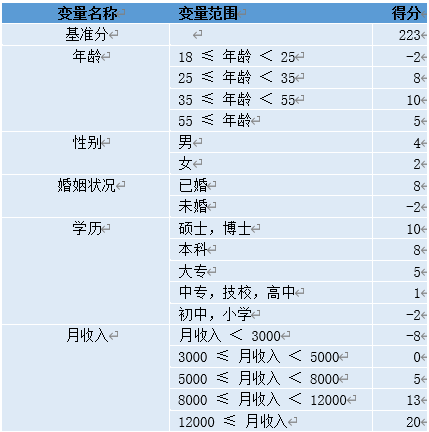## 如何搭信用评分卡模型？

• 变量特征是如何选取的？
• 特征的变量范围是如何进行划分的？
• 每个字段的分值是如何设定的？

### 变量选择

• 剔除跟目标变量不太相关的特征
• 消除由于线性相关的变量，避免特征冗余
• 减轻后期验证、部署、监控的负担
• 保证变量的可解释性

#### 单变量筛选

• WOE的取值范围是[-∞,+∞]，当分箱中好坏客户比例等于整体好坏客户比例时，WOE为0。
• 对于变量的一个分箱，这个分组的好坏客户比例与整体好坏客户比例相差越大，IV值越大，否则，IV值越小。
• IV值的取值范围是[0,+∞)，当分箱中只包含好客户或坏客户时，IV = +∞，当分箱中好坏客户比例等于整体好坏客户比例时，IV为0。

WOE（Weight of Evidence）叫做证据权重，WOE在业务中常有哪些应用呢？

• 处理缺失值：当数据源没有100%覆盖时，那就会存在缺失值，此时可以把null单独作为一个分箱。这点在分数据源建模时非常有用，可以有效将覆盖率哪怕只有20%的数据源利用起来。
• 处理异常值：当数据中存在离群点时，可以把其通过分箱离散化处理，从而提高变量的鲁棒性（抗干扰能力）。例如，age若出现200这种异常值，可分入“age > 60”这个分箱里，排除影响。
• 业务解释性：我们习惯于线性判断变量的作用，当x越来越大，y就越来越大。但实际x与y之间经常存在着非线性关系，此时可经过WOE变换。

$$WOE_i = ln(\frac{Bad_i}{Bad_T}/\frac{Good_i}{Good_T}) = ln(\frac{Bad_i}{Bad_T})-ln(\frac{Good_i}{Good_T})$$

IV（Information Value）是与WOE密切相关的一个指标，常用来评估变量的预测能力。因而可用来快速筛选变量。在应用实践中，其评价标准如下：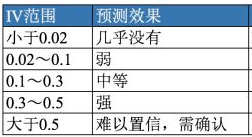$$IV_i = (\frac{Bad_i}{Bad_T}-\frac{Good_i}{Good_T}) * WOE_i$$

$$IV = \sum_{i=1}^{n}IV_i$$

• 前向选择forward：逐步将变量一个一个放入模型，并计算相应的指标，如果指标值符合条件，则保留，然后再放入下一个变量，直到没有符合条件的变量纳入或者所有的变量都可纳入模型。
• 后向选择backward：一开始将所有变量纳入模型，然后挨个移除不符合条件的变量，持续此过程，直到留下所有最优的变量为止。
• 逐步选择stepwise：该算法是向前选择和向后选择的结合，逐步放入最优的变量、移除最差的变量。

L1正则化通常称为Lasso正则化，它是在代价函数上增加了一个L1范数。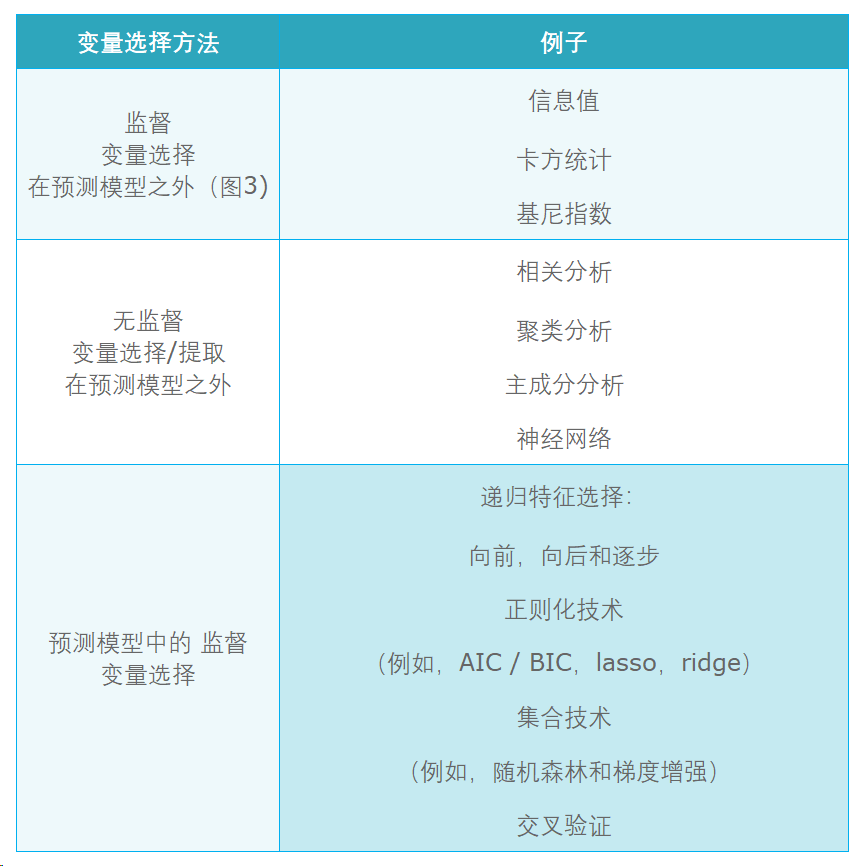• 变量两两相关性分析
• 变量的多重共线性分析

### 变量分箱

• 对连续变量进行分段离散化
• 将多状态的离散变量进行合并，减少离散变量的状态数

• 等频分箱：把自变量按从小到大的顺序排列，根据自变量的个数等分为k部分，每部分作为一个分箱。
• 等距分箱：把自变量按从小到大的顺序排列，将自变量的取值范围分为k个等距的区间，每个区间作为一个分箱。
• 聚类分箱：用k-means聚类法将自变量聚为k类，但在聚类过程中需要保证分箱的有序性。

• Split 分箱是一种自上而下(即基于分裂)的数据分段方法。Split 分箱和决策树比较相似，切分点的选择指标主要有 Entropy，Gini 指数和 IV 值等。
• Merge 分箱，是一种自底向上(即基于合并)的数据离散化方法。Merge 分箱常见的类型为Chimerge分箱。

ChiMerge 分箱

ChiMerge 分箱是目前最流行的分箱方式之一，其基本思想是如果两个相邻的区间具有类似的类分布，则这两个区间合并；否则，它们应保持分开。Chimerge通常采用卡方值来衡量两相邻区间的类分布情况。

ChiMerge的具体算法如下：

1. 输入：分箱的最大区间数n
2. 初始化
• 连续值按升序排列，离散值先转化为坏客户的比率，然后再按升序排列
• 为了减少计算量，对于状态数大于某一阈值 (建议为100) 的变量，利用等频分箱进行粗分箱
• 若有缺失值，则缺失值单独作为一个分箱
1. 合并区间
• 计算每一对相邻区间的卡方值
• 将卡方值最小的一对区间合并
• 重复以上两个步骤，直到分箱数量不大于n
1. 分箱后处理
• 对于坏客户比例为 0 或 1 的分箱进行合并 (一个分箱内不能全为好客户或者全为坏客户)。
• 对于分箱后某一箱样本占比超过 95% 的箱子进行删除。
• 检查缺失分箱的坏客户比例是否和非缺失分箱相等，如果相等，进行合并。
1. 输出：分箱后的数据和分箱区间。

• 特征分箱可以有效处理特征中的缺失值和异常值。
• 特征分箱后，数据和模型会更稳定。
• 特征分箱可以简化逻辑回归模型，降低模型过拟合的风险，提高模型的泛化能力。
• 将所有特征统一变换为类别型变量。
• 分箱后变量才可以使用标准的评分卡格式，即对不同的分段进行评分。

### WOE编码

• Numerical Variable，数值变量。例如逾期金额，天数
• Categorical Variable，类别变量。例如客户职业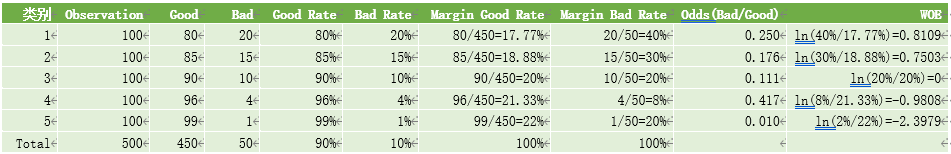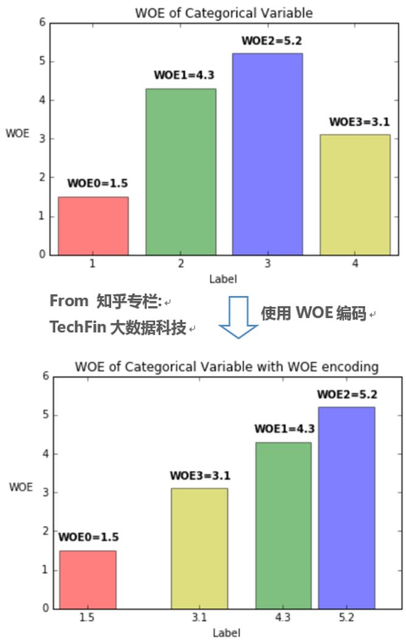• 可提升模型的预测效果
• 将自变量规范到同一尺度上
• WOE能反映自变量取值的贡献情况
• 有利于对变量的每个分箱进行评分
• 转化为连续变量之后，便于分析变量与变量之间的相关性
• 与独热向量编码相比，可以保证变量的完整性，同时避免稀疏矩阵和维度灾难

### 模型训练

Logistic回归是信用评分中用于解决二元分类问题的常用技术。逻辑回归通过sigmoid函数$y = \frac{1}{1+e^{-z}}$ 将线性回归模型$z=\boldsymbol{w}^T\boldsymbol{x}+b$产生的预测值转换为一个接近0或1的拟合值：

$$h(x)=\frac{1}{1+e^{-z}}=\frac{1}{1+e^{-(\boldsymbol{w}^T\boldsymbol{x}+b)}}$$

$$Odds = \frac{p}{1-p}$$

$$p = \frac{Odds}{1+Odds}$$

$$Score = A – B\log(Odds)$$

$$log(Odds)=\beta _0 + \beta _1x_1+…+\beta _nx_n$$

• 给某个特定的比率设定特定的预期分值
• 确定比率翻番的分数（PDO） 根据以上的分析，我们首先假设比率为x的特定点的分值为P。则比率为2x的点的分值应该为P+PDO。

$$P = A – B\log(x)$$

$$P – PDO = A – B\log(2x)$$

$$Score = 6.78 -14.43\log(Odds)$$

$$Score = A – B\{\beta _0+\beta _1x_1+…+\beta _nx_n\}$$

$$Score = A-B\{\beta _0+(\beta _1\omega _{11})\delta _{11}+(\beta _1\omega _{12})\delta _{12}+…+(\beta _2\omega _{21})\delta _{21}+…\}$$

• $\omega _{ij}$为第i行第j个变量的WOE，为已知变量
• $\beta _i$为逻辑回归方程中的系数，为已知变量
• $\delta _{ij}$为二元变量，表示变量i是否取第j个值。

$$Score = (A-B\beta _0)-(B\beta _1\omega _{11})\delta _{11}-(B\beta _1\omega _12)\delta _{12}-…-(B\beta _x\omega _{x1}-…$$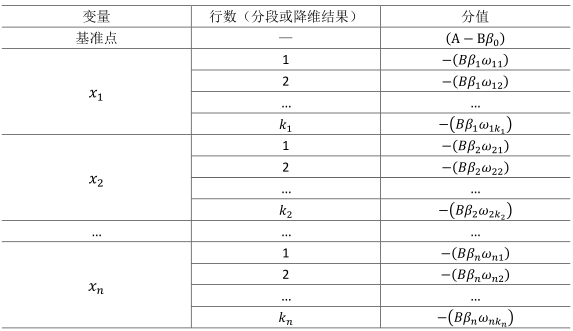#### 拒绝推断

• 简单赋值法：人为指定被拒绝账户的标签
• 忽略被拒绝申请
• 所有被拒申请赋值为违约标签
• 按比例赋值，使得其坏客户率是通过样本的2~5倍以上
• 强化法：通过外推法确定拒绝账户的标签
• 简单强化法：使用通过客户开发的模型对被拒绝客户评分，将其中低分段赋予违约标签。使得拒绝客户的坏客户率为通过的2~5倍以上
• 模糊强化法：通过模型计算得到正常和违约概率。
• 打包强化法：先用开发的评分卡对被拒客户评分，然后指定每个分值区间的违约客户数量。

#### 模型表现

• 冠军挑战者选择最佳表现模式的方法
• 在看不见的数据上测试模型性能并将其与训练数据进行比较
• 选择最佳阈值，最大化真阳性率，同时最小化假阳性率

ROC曲线下面积（AUC）是指示分类器预测能力的有用度量。在信用风险中，0.75或更高的AUC是行业认可的标准和模型验收的先决条件。

• 增加客户数量
• 减少风险损失
• 最大化利润

• 评分临界值：实现通过率、坏客户率、或利润损失率等业务目标
• 通过交叉决策矩阵实现风险定价，实现差异化的利率、额度等：
• 风险评分与利润损失比
• 风险评分与债务收入比
• 风险评分与流失倾向评分

• 在进行数据描述时变量应该有意义。通常，某些变在特定客群的不同风险模型中重复出现。例如，信用卡行为评分卡模型中，授信使用率经常出现；申请评分卡模型中收入水平、职业和历史信贷产品拥有情况比人口统计变量重要。
• 变量的预测力或贡献度，应该在模型的变量之间分布。
• 模型中不应该包含太多变量。通常，包含的变量不超过9~20个(最优10~12个)。变量太多可能导致过拟合，变量太少往往区分度不够。
• 最终模型的变量应该能够确保包含稳健一致的数据，并在后续实施阶段能够准确获取。

## 评分卡模型搭建实战

 列名 字段说明 SeriousDlqin2yrs 两年内是否有严重违约（好坏用户判断） RevolvingUtilizationOfUnsecuredLines 可用信贷额度比例，信用卡和个人信用额度（不动产和汽车贷款等分期付款债务除外）的总余额除以信用额度之和 age 借款人年龄 NumberOfTime30-59DaysPastDueNotWorse 两年内35-59天逾期次数 DebtRatio 借款人负债比率（每月债务支付、赡养费、生活费之和除以月收入） MonthlyIncome 借款人月收入 NumberOfOpenCreditLinesAndLoans 开放式信贷和贷款数量 NumberOfTimes90DaysLate 两年内90天或高于90天逾期的次数 NumberRealEstateLoansOrLines 不动产贷款或额度数量 NumberOfTime60-89DaysPastDueNotWorse 两年内60-89天逾期次数 NumberOfDependents 借款人家属数量（不包括本人在内）

### 探索数据

import pandas as pd
from dataprep.eda import plot
import warnings
warnings.filterwarnings('ignore')

train_data.columns = ['严重违约', '可用额度比例','年龄', '35-69天逾期次数', '负债比例','月收入','普通贷款数量','高于90天逾期次数','不动产贷款数量','60-89天逾期次数','家属数量']
train_data = train_data[['年龄','家属数量','月收入','负债比例','可用额度比例','普通贷款数量','不动产贷款数量','35-69天逾期次数','60-89天逾期次数','高于90天逾期次数','严重违约']]

# 手工探索数据
print(train_data.shape)
print(train_data.info())
print(train_data.isnull().sum())
print(train_data.describe().T)
print(train_data['严重违约'].value_counts())
print(train_data['严重违约'].sum()/train_data['严重违约'].count())

# 使用EDA工具探索数据
plot(train_data)


### 数据预处理

1）缺失值处理

• 直接删除含有缺失值的样本
• 根据样本之间的相似性填补缺失值
• 根据变量之间的相关关系填补缺失值

from sklearn.ensemble import RandomForestRegressor

def fill_income_missing(data, to_fill):
df = data.copy()
columns = [*df.columns]
columns.remove(to_fill)

# 移除有缺失值的列
columns.remove('家属数量')
X = df.loc[:, columns]
y = df.loc[:, to_fill]
X_train = X.loc[df[to_fill].notnull()]
y_train = y.loc[df[to_fill].notnull()]
X_pred = X.loc[df[to_fill].isnull()]
rfr = RandomForestRegressor(random_state=22, n_estimators=200, max_depth=3, n_jobs=-1)
rfr.fit(X_train, y_train)
y_pred = rfr.predict(X_pred).round()
df.loc[df[to_fill].isnull(), to_fill] = y_pred
return df

def fill_dependents_missing(data, to_fill):
df = data.copy()
columns = [*df.columns]
columns.remove(to_fill)

X = df.loc[:, columns]
y = df.loc[:, to_fill]
X_train = X.loc[df[to_fill].notnull()]
y_train = y.loc[df[to_fill].notnull()]
X_pred = X.loc[df[to_fill].isnull()]
rfr = RandomForestRegressor(random_state=22, n_estimators=200, max_depth=3, n_jobs=-1)
rfr.fit(X_train, y_train)
y_pred = rfr.predict(X_pred).round()
df.loc[df[to_fill].isnull(), to_fill] = y_pred
return df

train_data = fill_income_missing(train_data, '月收入')
train_data = fill_dependents_missing(train_data, '家属数量')
print(train_data.isnull().sum())


2) 异常值处理

a. 删除年龄为0的数据

train_data = train_data.loc[train_data['年龄'] > 0]

b. 去除逾期次数中的异常数据

import matplotlib.pyplot as plt

columns = ['35-69天逾期次数','60-89天逾期次数','高于90天逾期次数']
train_data.loc[:, columns].plot.box(vert=False)

train_data = train_data[(train_data['35-69天逾期次数'] < 90) & (train_data['60-89天逾期次数'] < 90)  & (train_data['高于90天逾期次数'] < 90)]


### 信用卡模型训练

1） 特征离散化（ChiMerge）

from scorecardbundle.feature_discretization import ChiMerge as cm
from scorecardbundle.feature_discretization import FeatureIntervalAdjustment as fia
from scorecardbundle.feature_encoding import WOE as woe
from scorecardbundle.feature_selection import FeatureSelection as fs
from scorecardbundle.model_training import LogisticRegressionScoreCard as lrsc
from scorecardbundle.model_evaluation import ModelEvaluation as me

X = train_data.iloc[:, :-1]
y = train_data.iloc[:, -1]

from sklearn.model_selection import train_test_split
X_train, X_test, y_train, y_test = train_test_split(X, y, random_state=0, test_size=0.25)

trans_cm = cm.ChiMerge(max_intervals=10, min_intervals=5, output_dataframe=True)
result_cm = trans_cm.fit_transform(X_train, y_train)
print(trans_cm.boundaries_) # 每个特征的区间切分


2） 特征编码（WOE）和评估（IV）

trans_woe = woe.WOE_Encoder(output_dataframe=True)
result_woe = trans_woe.fit_transform(result_cm, y_train)
print(trans_woe.iv_) # 每个特征的信息值 (iv)
print(trans_woe.result_dict_) # 每个特征的WOE字典和信息值 (iv)


3) 手动调整分箱

col = '年龄'
fia.plot_event_dist(result_cm[col],y_train,x_rotation=60)
new_x = cm.assign_interval_str(X_train[col].values,[22, 33, 43, 53, 62, 67, 74]) # apply new interval boundaries to the feature
woe.woe_vector(new_x, y_train.values) # check the information value of the resulted feature that applied the new intervals
fia.plot_event_dist(new_x,y_train, x_label=col,x_rotation=60)

feature_list = []
result_cm[col] = new_x # great explainability and predictability. Select.
feature_list.append(col)
print(feature_list)


4) WOE编码

trans_woe = woe.WOE_Encoder(output_dataframe=True)
result_woe = trans_woe.fit_transform(result_cm[feature_list], y_train)
print(trans_woe.iv_)


5) 特征选择

fs.selection_with_iv_corr(trans_woe, result_woe) # corr_with 列示了与该特征相关性过高的特征和相关系数

6) 模型训练

model = lrsc.LogisticRegressionScoreCard(trans_woe, PDO=-20, basePoints=100, verbose=True)
model.fit(result_woe, y_train)
print(model.woe_df_) # 从woe_df_属性中可得评分卡规则


7) 模型校验

sc_table = model.woe_df_.copy()
result = model.predict(X_train[feature_list], load_scorecard=sc_table) # Scorecard should be applied on the original feature values
result_test = model.predict(X_test[feature_list], load_scorecard=sc_table) # Scorecard should be applied on the original feature values
result.head() # if model object's verbose parameter is set to False, predict will only return Total scores

# Train
evaluation = me.BinaryTargets(y_train, result['TotalScore'])
evaluation.plot_all()

# Validation
evaluation = me.BinaryTargets(y_test, result_test['TotalScore'])
evaluation.plot_all()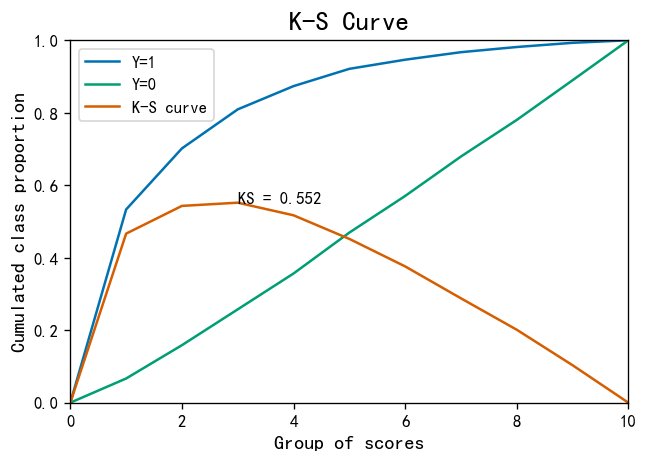KS指标: 用以评估模型对好、坏客户的判别区分能力，计算累计坏客户与累计好客户百分比的最大差距。KS值范围在0%-100%，判别标准如下：

• KS: <20% : 差
• KS: 20%-40% : 一般
• KS: 41%-50% : 好
• KS: 51%-75% : 非常好
• KS: >75% : 过高，需要谨慎的验证模型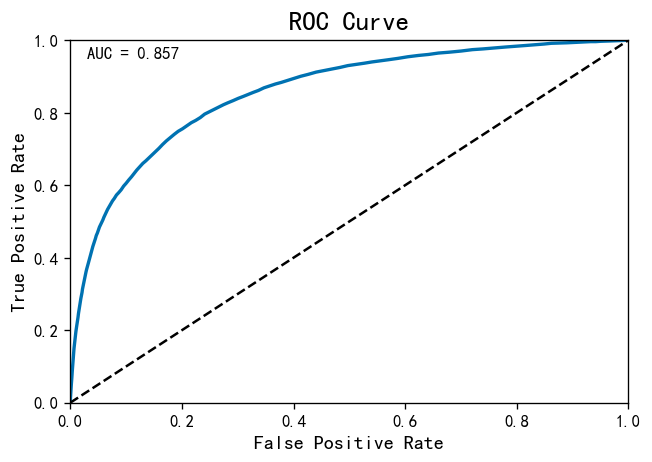ROC曲线就越往左上方靠拢，它下面的面积(AUC)也就越大：

• 如果AUC的值达到80，那说明分类器分类非常准确
• 如果AUC值在60～0.80之间，那分类器有优化空间，可以通过调节参数得到更好的性能
• 如果AUC值小于60，那说明分类器模型效果比较差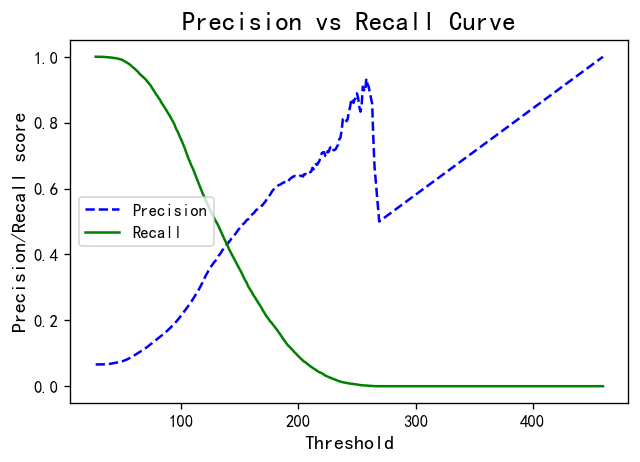- - 标点符

- - 美团点评技术团队

- - PingWest品玩

## 模型制作

- 小鱼儿 - 非正常人类研究中心 – Mtime时光网
1.材料：一大袋的一次性筷子（花了60块钱）；5支502胶水；5张粗砂纸；记号笔一只；锋利的美工刀片若干，破剪刀一把. 就是这种屌毛筷子，质量也太他妈的差了点，80%都是弯的 . 随便提一下：我的脚丫子还是蛮性感滴 . 开始动工了!!  先做门框跟房子的底架. 3.不好意思，忘了交代一下了，我是先画图纸的，看到那张纸了没有.

## MapReduce编程模型

- - CSDN博客云计算推荐文章

## 关于BOM模型

- - CSDN博客编程语言推荐文章

## 对象的消息模型

- loudly - 酷壳 - CoolShell.cn
[ ———— 感谢 Todd 同学 投递本文，原文链接 ———— ]. 话题从下面这段C++程序说起，你认为它可以顺利执行吗. 试试的确可以顺利运行输出hello world，奇怪吗. 其实并不奇怪，根据C++对象模型，类的非虚方法并不会存在于对象内存布局中，实际上编译器是把Hello方法转化成了类似这样的全局函数：.

## JS三维模型库 Three.js

- Le - 开源中国社区最新软件
Three.js 是一款运行在浏览器中的 3D 引擎，你可以用它创建各种三维场景，包括了摄影机、光影、材质等各种对象. 你可以在它的主页上看到许多精采的演示. 不过，这款引擎目前还处在比较不成熟的开发阶段，其不够丰富的 API 以及匮乏的文档增加了初学者的学习难度（尤其是文档的匮乏） 演示：http://mrdoob.github.com/three.js/.

- - NoSQLFan

- - ImportNew